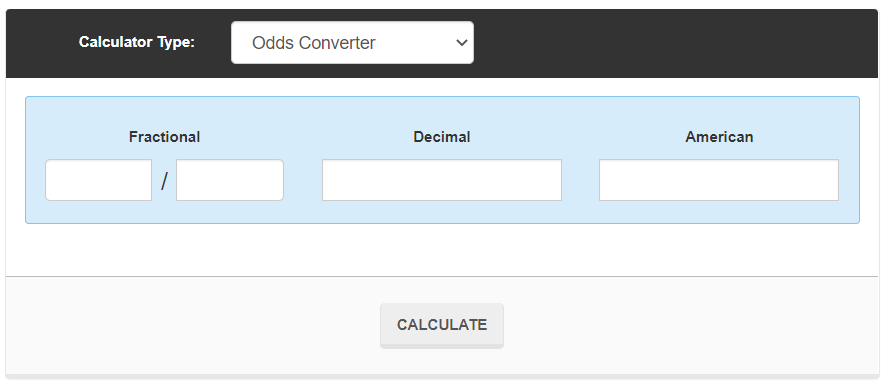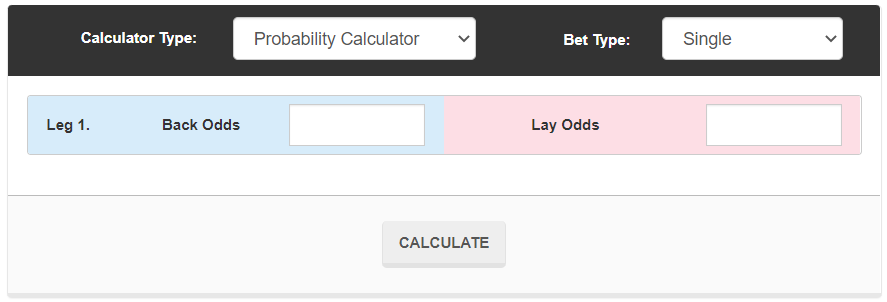# Betting Odds Converter And Probability Calculator

## Betting Odds Converter

The Odds Converter Calculator is a simple tool that allows you to quickly convert bookmaker odds between fractional, decimal and American odds. This can be really handy for bookmakers that don’t allow you to change the odds format.

Without access to a betting odds conversion calculator you would have to spend time manually converting the bookmakers’ odds.

Not only is the calculator faster than manually converting odds, but it also substantially reduces the chance of human error. Instead of double or triple checking your entire calculation, all you have to do is make sure you typed the correct numbers into the calculator - that’s it!## Probability Calculator

The Probability Calculator can be found by changing the Calculator Type from Odds Converter to Probability Calculator on the calculator above.

The Probability Calculator is a handy tool for understanding how likely your bets are to win.

You just need to enter the back and lay odds of your chosen bet, and the calculator will then tell you the implied probability of your bet winning.

This tools works for both single bets, or accumulators, like doubles, trebles and fourfolds.betting-odds-probability-calculator.png

## How To Convert Betting Odds Manually Without A Calculator

The Outplayed betting odds converter is a quick, simple tool to convert fractional, decimal and American odds. If you wish to convert betting odds yourself, follow these methods:

### Convert Decimal Odds To Fractional Odds

Subtract 1.00 and then find the nearest whole integer (so for example 3.75 - 1.00 becomes 2.75/1, or 11/4).

### Convert US Odds To Decimal Odds

If the US odds are positive, divide by 100 and add 1. If it is negative, divide 100 by the US odds (without the minus sign) and add 1.

### Convert Fractional Odds To Decimal Odds

Divide the first figure by the second figure add 1.00 (so 11/4 = 2.75, then add 1.00 = 3.75).

## Interested In Making Money From Sportsbook Free Bets, Price Boosts And More?

Outplayed is the UK's largest matched betting community.

We've shown over 150,000 people how to make a side income by turning bookie free bets, price boosts and other special offers into extra cash!

You can start learning with our free trial to make your first profits